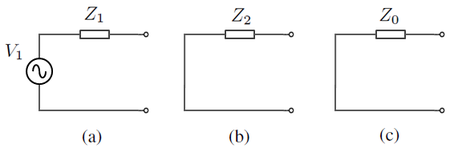# Sequence NetworksReduced sequence networks: (a) Positive sequence, (b) Negative sequence, (c) Zero sequence

In symmetrical component analysis (e.g. for unbalanced faults), a balanced three-phase electrical network can be broken down into three sequence networks, which are independent, de-coupled sub-networks comprising only quantities in the same sequence, i.e. the positive sequence network contains only positive sequence quantities, the negative sequence network contains only negative sequence quantities and the zero sequence network contains only zero sequence quantities.

For any location in the system, the sequence networks can be reduced to Thevenin equivalent circuits (see the figure to the right). Notice that only the positive sequence network has a voltage source. This is because in a balanced system, there are no negative sequence or zero sequence voltages.

## Constructing Sequence Networks

Sequence networks are constructed from two-port sequence networks of individual elements. This is best illustrated by example. Given the system below:

The individual positive, negative and zero sequence networks for each of the network elements are shown in the figure below:

These individual networks are then connected together to form the positive, negative and zero sequence networks for the overall system:

## Reduction of Sequence Networks

At any location in the system, the overall sequence networks developed in the previous section can be reduced to a Thevenin equivalent circuit (positive sequence) or equivalent impedances (negative and zero sequence).

For example, consider a fault on the grid side of cable C1 as follows:

For this fault location, we can represent the sequence networks as reduced sequence networks:

where

$Z_{1}=\left[\left(X_{d}^{''}+X_{1,T1}\right)||Z_{1,L1}+Z_{1,C}\right]||\left(X_{1,T2}+X_{1,S}\right)||Z_{1,L2}\,$$Z_{2}=\left[\left(X_{2,G1}+X_{2,T1}\right)||Z_{2,L1}+Z_{2,C}\right]||\left(X_{2,T2}+X_{2,S}\right)||Z_{2,L2}\,$$Z_{0}=\left(X_{0,T1}||Z_{0,L1}+Z_{0,C}\right)||\left(X_{0,T2}+X_{0,S}\right)||Z_{0,L2}\,$